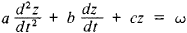# Modeling, Analog

The following article is from The Great Soviet Encyclopedia (1979). It might be outdated or ideologically biased.

## Modeling, Analog

(also analog simulation), one of the most important kinds of modeling; based on the analogy (more accurately, the isomorphism) of phenomena that differ in physical nature but are described by the same differential, algebraic, or any other mathematical equations.

A simple example is two systems, the first of which is mechanical and consists of a shaft that transmits rotation through a spring to a spindle rigidly connected to a flywheel that is partially immersed in a viscous damping fluid. The second system is electrical and consists of a source of electromotive force that is connected through an inductance coil, a capacitor, and a resistor to a kilowatt-hour meter. If the values of inductance, capacitance, and resistance are chosen such that they correspond in a specific manner to the inertia of the flywheel, the elasticity of the spring, and the friction of the fluid, the systems then will display a structural and functional similarity (even an identity) that means, among other things, that they will be described by the same differential equation with constant coefficients of the typeThis equation may be a “theoretical model” for both systems, either of which may be the “experimental model” for this equation and the “analog model” for the other system. This analogy is the basis for the electrical modeling of mechanical systems: electrical models are far more convenient than mechanical systems for experimental study.

Another traditional area of application for analog modeling is the study of thermal conductivity based on electrothermal and hydrothermal analogies. In the first case the analogs of the temperature field and heat capacity in a solid body are the electric potential field in an electrically conducting medium and the capacitances of several capacitors, respectively; in the second case the temperature is simulated by the water level in vertical glass vessels that form the hydraulic model, the heat capacity of an elementary volume is simulated by the cross-sectional area of the vessels, and the thermal resistance is simulated by the hydraulic resistance of the tubes that connect the vessels.

The method of luminous simulation, in which the fluxes of thermal radiation are replaced by similar fluxes of luminous radiation, is often used to study radiant heat transfer. In this way the angular coefficients of the radiation are measured, and if the optical properties (degree of blackness and absorptivity) of the corresponding surfaces in the model and in nature are identical, the distribution of the thermal fluxes over the surfaces in the radiant heat-transfer system are also determined.

Before the development of digital computers in the late 1940’s, analog modeling was the main method of “object-mathematical modeling” for any processes dealing with the propagation of electromagnetic and acoustic waves, the diffusion of gases and fluids, the movement and filtration of fluids in porous media, and the torsion of rods (in view of this it was then often called simply mathematical modeling), and for each specific modeling problem a special “network” model was constructed (using various electrical impedance elements interconnected to form a two-dimensional circuit grid). Analog computers made possible the modeling of entire classes of similar problems. At the present time the importance of analog modeling has been greatly reduced, because modeling on digital computers has great advantages with respect to accuracy and versatility. For sufficiently specific and specialized problems, analog modeling has its advantages (the simplicity and consequent inexpensiveness of the technology). The two methods are also frequently used in combination.

The Great Soviet Encyclopedia, 3rd Edition (1970-1979). © 2010 The Gale Group, Inc. All rights reserved.
Site: Follow: Share:
Open / Close